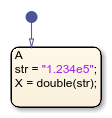double

Convert string to double precision value

Description

example

X = double(str) converts the text in string str to a double-precision value.

str contains text that represents a number. Text that represents a number can contain:

• Digits

• A decimal point

• A leading + or - sign

• An e preceding a power of 10 scale factor

If double cannot convert the text to a number, it returns a NaN value.

Note

Only Stateflow® charts that use MATLAB® as the action language support the double operator.

Examples

expand all

Return a value of -3.145.

h = "-3.145"
x = double(h)Limitations